## 在rmarkdown中编写数学公式

R的极客理想系列文章，涵盖了R的思想，使用，工具，创新等的一系列要点，以我个人的学习和体验去诠释R的强大。

R语言作为统计学一门语言，一直在小众领域闪耀着光芒。直到大数据的爆发，R语言变成了一门炙手可热的数据分析的利器。随着越来越多的工程背景的人的加入，R语言的社区在迅速扩大成长。现在已不仅仅是统计领域，教育，银行，电商，互联网….都在使用R语言。

• 张丹，分析师/程序员/Quant: R,Java,Nodejs
• blog: http://blog.fens.me
• email: bsspirit@gmail.com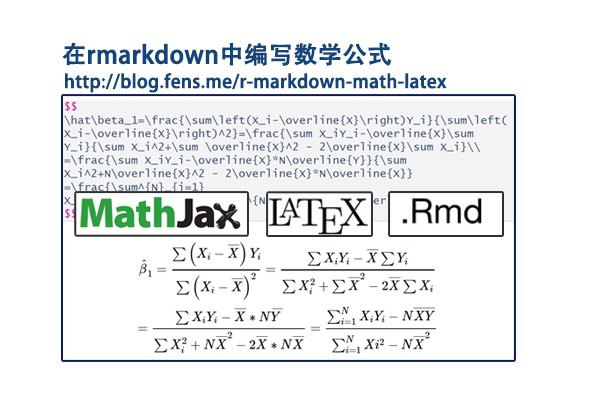1. 在rmarkdown文档中加入数学公式
2. laTex 数学公式语法
3. 用R来完成作业

## 1. 在rmarkdown文档中加入数学公式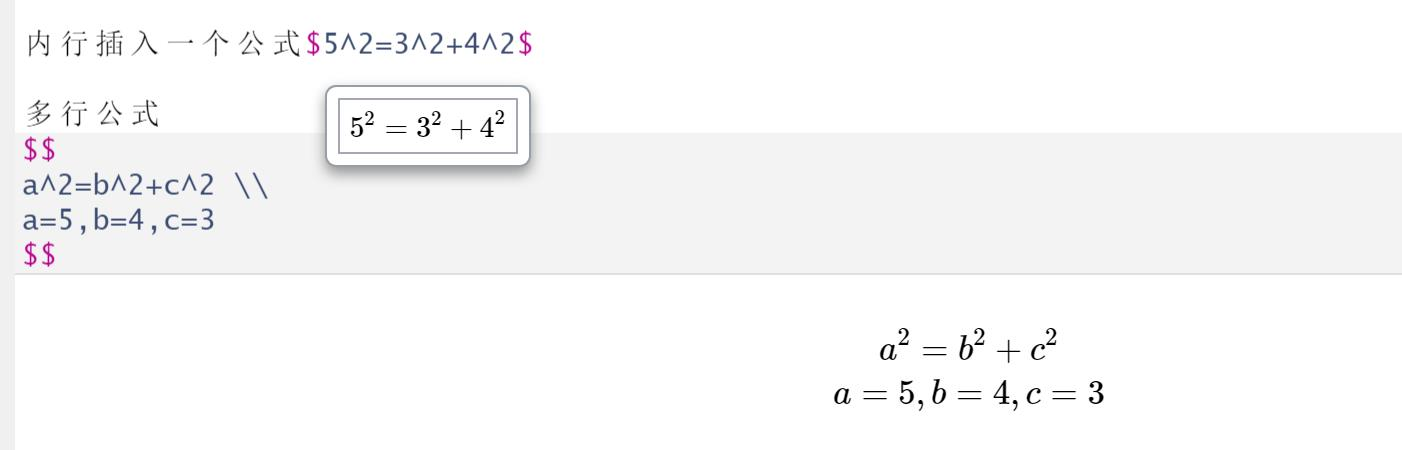## 2. laTex 数学公式语法

2.1 希腊字母表

alphaAAα\alpha
betaBBβ\beta
gammaΓ\Gammaγ\gamma
deltaΔ\Deltaδ\delta
epsilonEEϵ\epsilon
zetaZZζ\zeta
etaHHη\eta
thetaΘ\Thetaθ\theta
iotaIIι\iota
kappaKKκ\kappa
lambdaΛ\Lambdaλ\lambda
muMMμ\mu
nuNNν\nu
xiΞ\Xiξ\xi
omicronOOο\omicron
piΠ\Piπ\pi
rhoPPρ\rho
sigmaΣ\Sigmaσ\sigma
tauTTτ\tau
upsilonΥ\Upsilonυ\upsilon
phiΦ\Phiϕ\phi
chiXXχ\chi
psiΨ\Psiψ\psi
omegaΩ\Omegaω\omega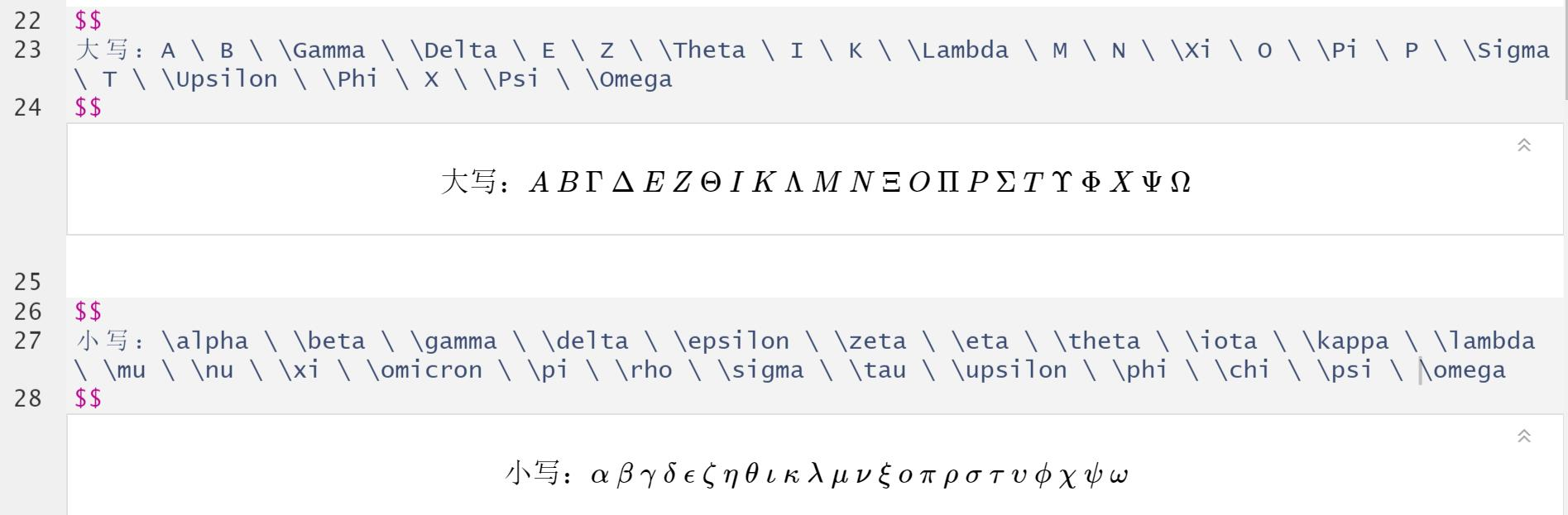2.2 四则运算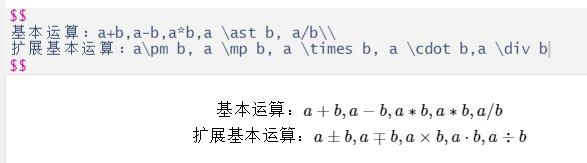2.3 括号

1、小括号与方括号

2、大括号

3、尖括号

4、上取整和下取整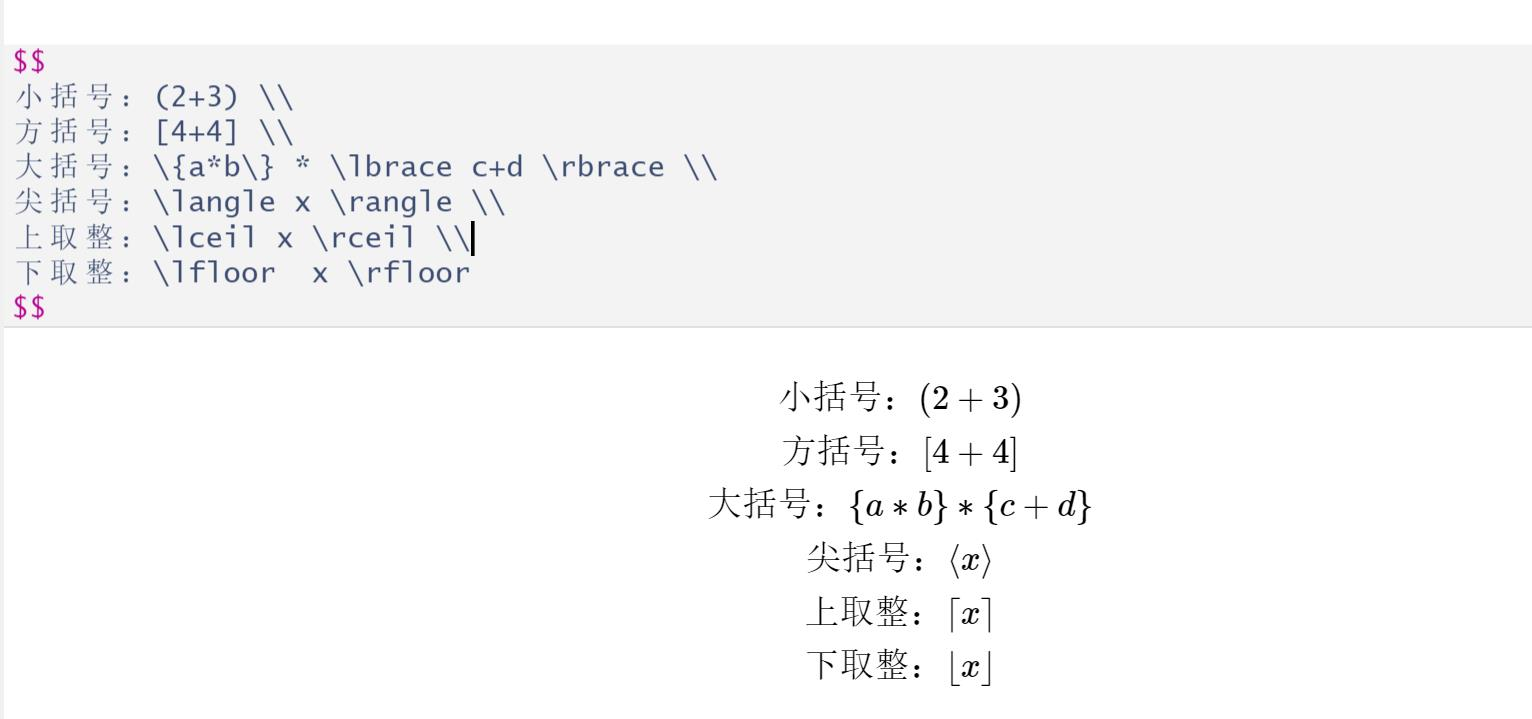2.4 上标、下标、顶部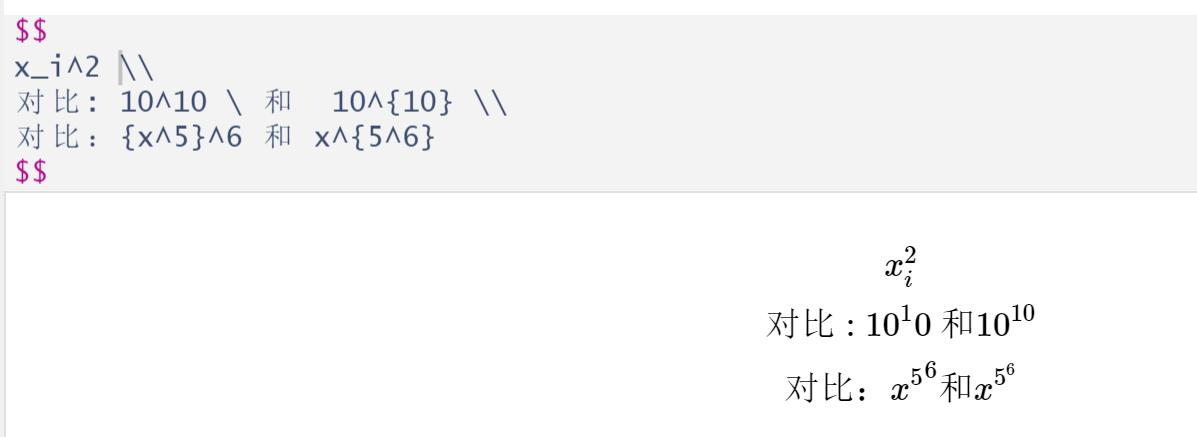2.5 求和，积分，连乘、交集并集、最大最小、自变量集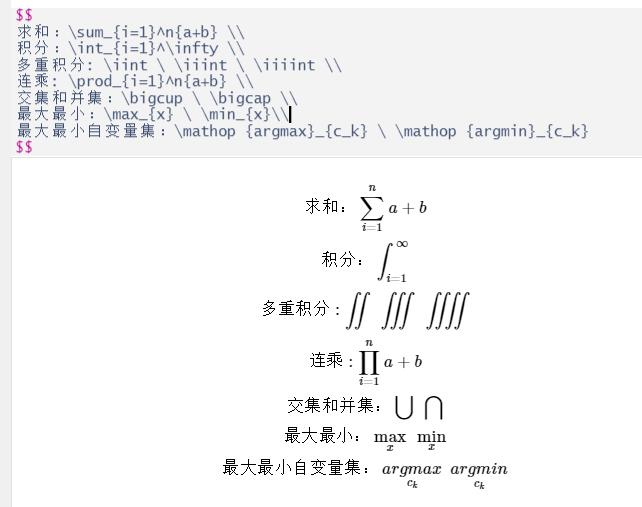2.6 分式、连分式、根式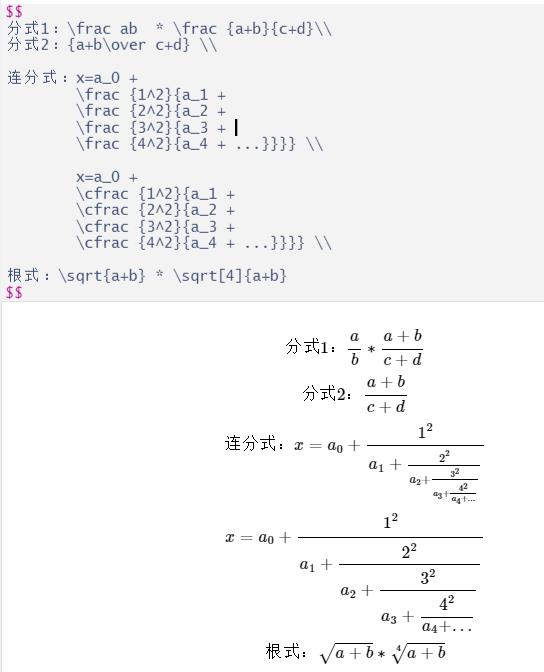2.7 分类表达式、多行表达式、方程组、占位符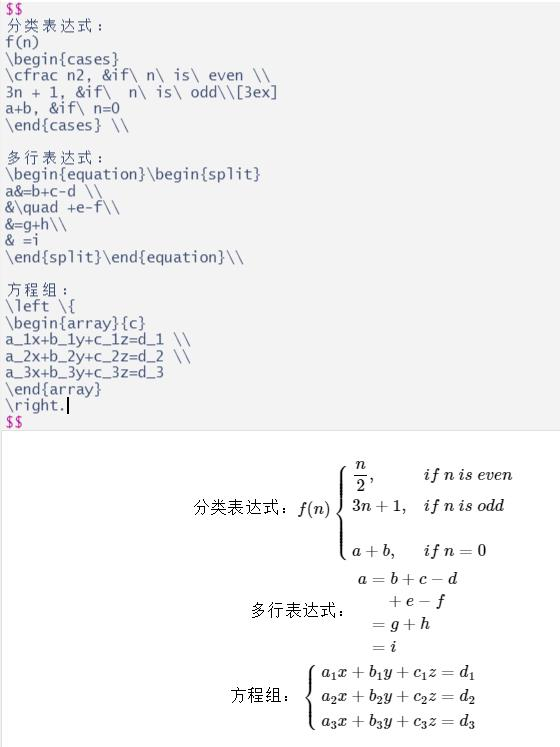2.8 三角函数，反三角函数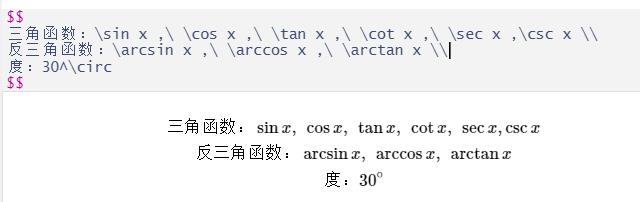2.9 运算符，排列组合，求模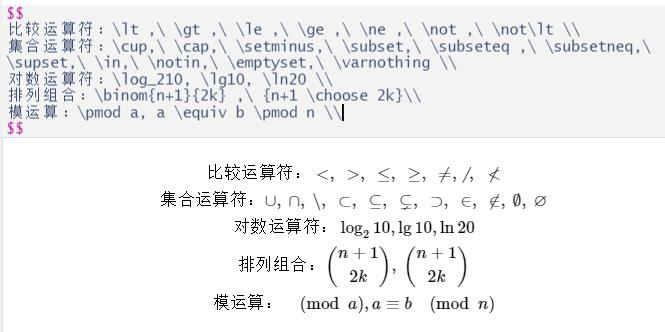2.10 特殊符号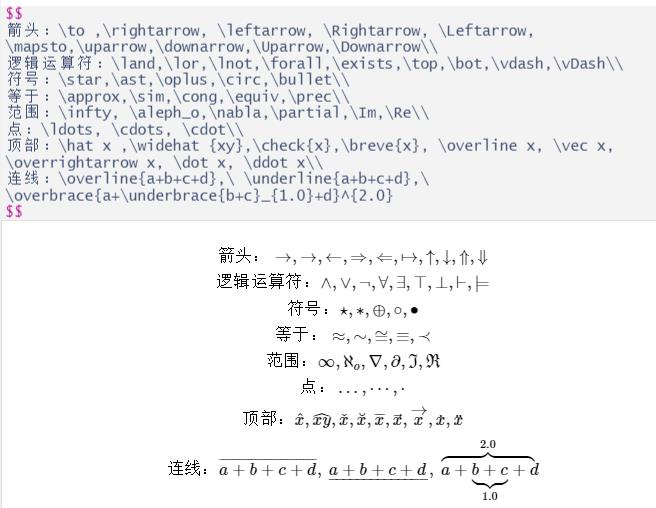2.11 表格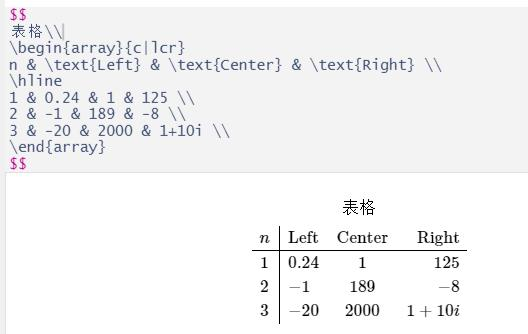2.12 矩阵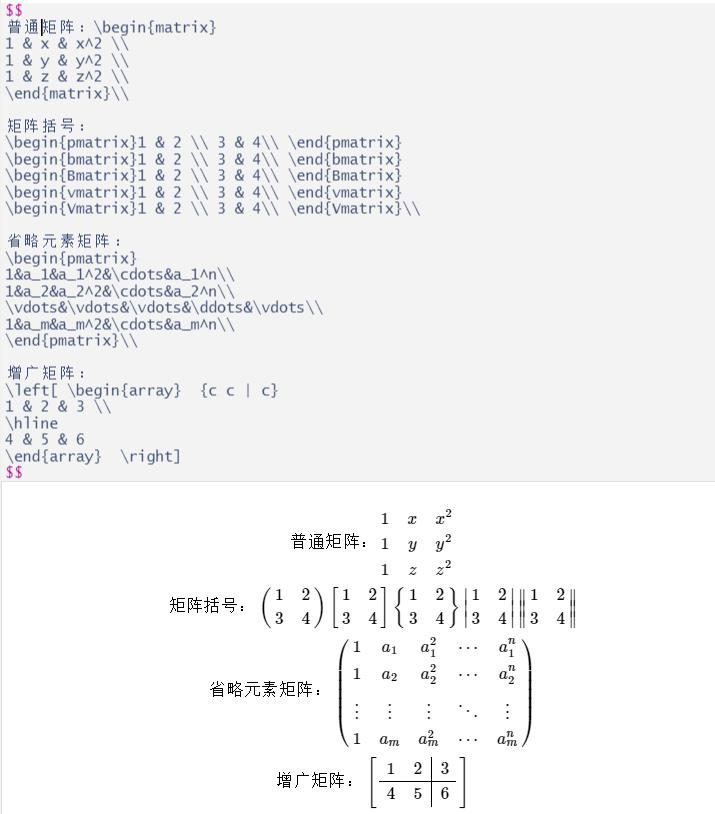2.13 标记与引用

bbbb

2.14 字体

Fraktur字母\mathfrak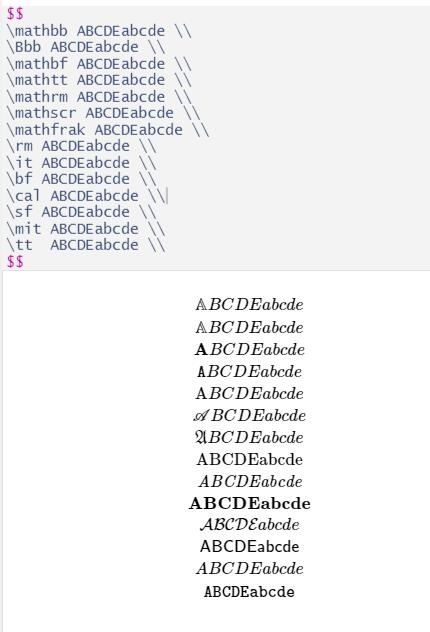2.15 颜色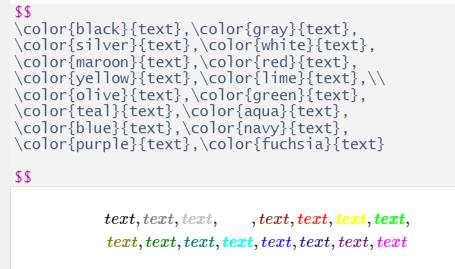## 3. 用R来完成作业

1. Show that the OLS estimator for β1 in the simple linear regression model can be written as.\hat\beta_1=\frac{\sum\left(X_i-\overline{X}\right)Y_i}{\sum\left(X_i-\overline{X}\right)^2}=\frac{\sum X_iY_i-\overline{X}\sum Y_i}{\sum X_i^2+\sum \overline{X}^2 - 2\overline{X}\sum X_i}\\
=\frac{\sum X_iY_i-\overline{X}*N\overline{Y}}{\sum X_i^2+N\overline{X}^2 - 2\overline{X}*N\overline{X}}
=\frac{\sum^{N}_{i=1} X_iY_i-N\overline{XY}}{\sum^{N}_{i=1}Xi^2-N\overline{X}^2}


2a.Find the OLS estimator for β1 in the linear regression model with no intercept term, i.e., in the regression Yi=β1Xi+Ei, i=1,2,...,N. Which algebraic properties of OLS estimatios discussed in the notes continue to hold and which are lost? Will the R^2 still necessarily lie between 0 and 1?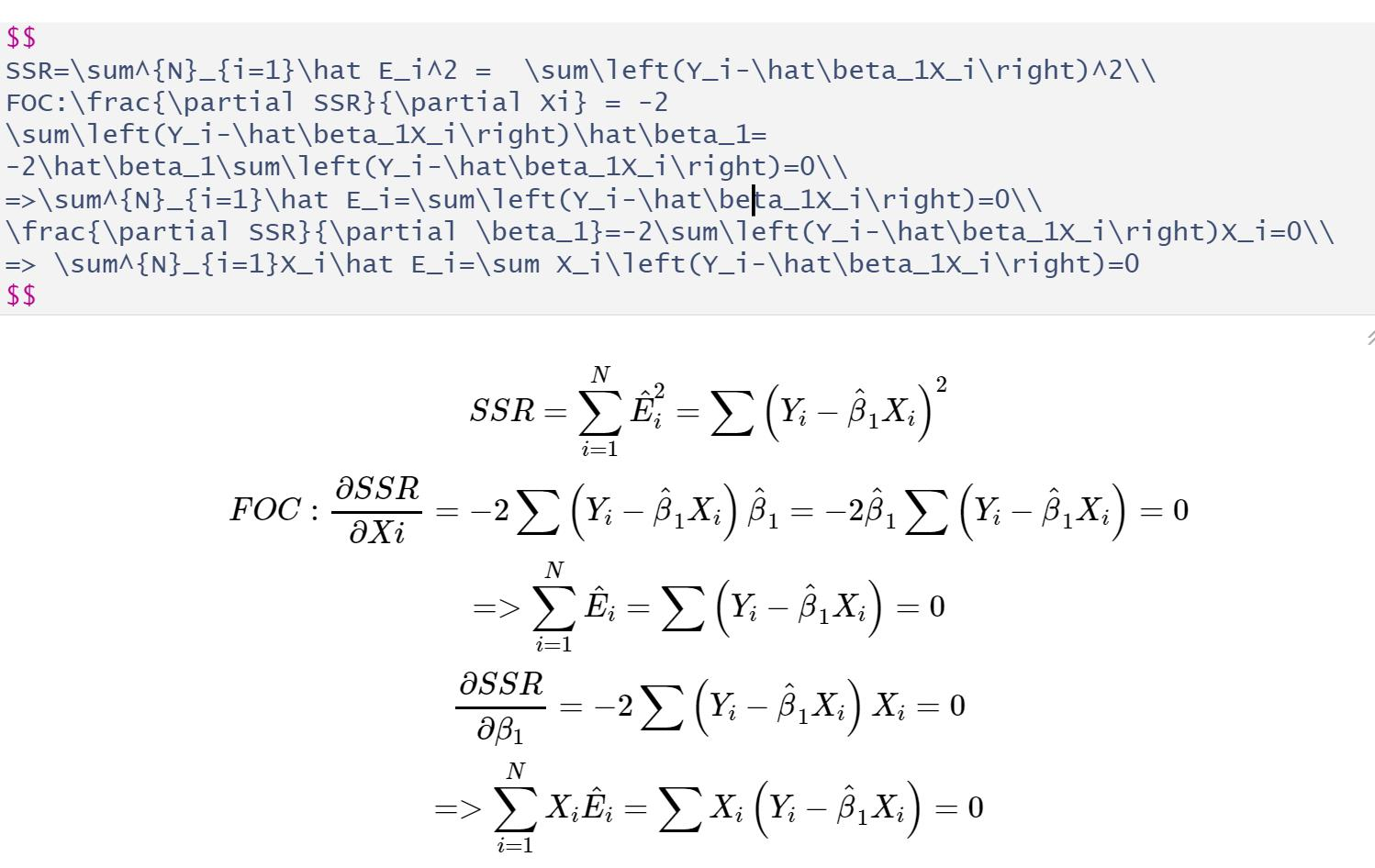SSR=\sum^{N}_{i=1}\hat E_i^2 =  \sum\left(Y_i-\hat\beta_1X_i\right)^2\\
FOC:\frac{\partial SSR}{\partial Xi} = -2 \sum\left(Y_i-\hat\beta_1X_i\right)\hat\beta_1= -2\hat\beta_1\sum\left(Y_i-\hat\beta_1X_i\right)=0\\
=>\sum^{N}_{i=1}\hat E_i=\sum\left(Y_i-\hat\beta_1X_i\right)=0\\
\frac{\partial SSR}{\partial \beta_1}=-2\sum\left(Y_i-\hat\beta_1X_i\right)X_i=0\\
=> \sum^{N}_{i=1}X_i\hat E_i=\sum X_i\left(Y_i-\hat\beta_1X_i\right)=0


2b. What is the OLS estimator for β0 in the linear regression model with no regressor, i.e., in the regression Yi=β0 + Ei? What is the R^2 for this regression? Remark :All of regressions will include the intercept term, unless explicitly stated otherwise.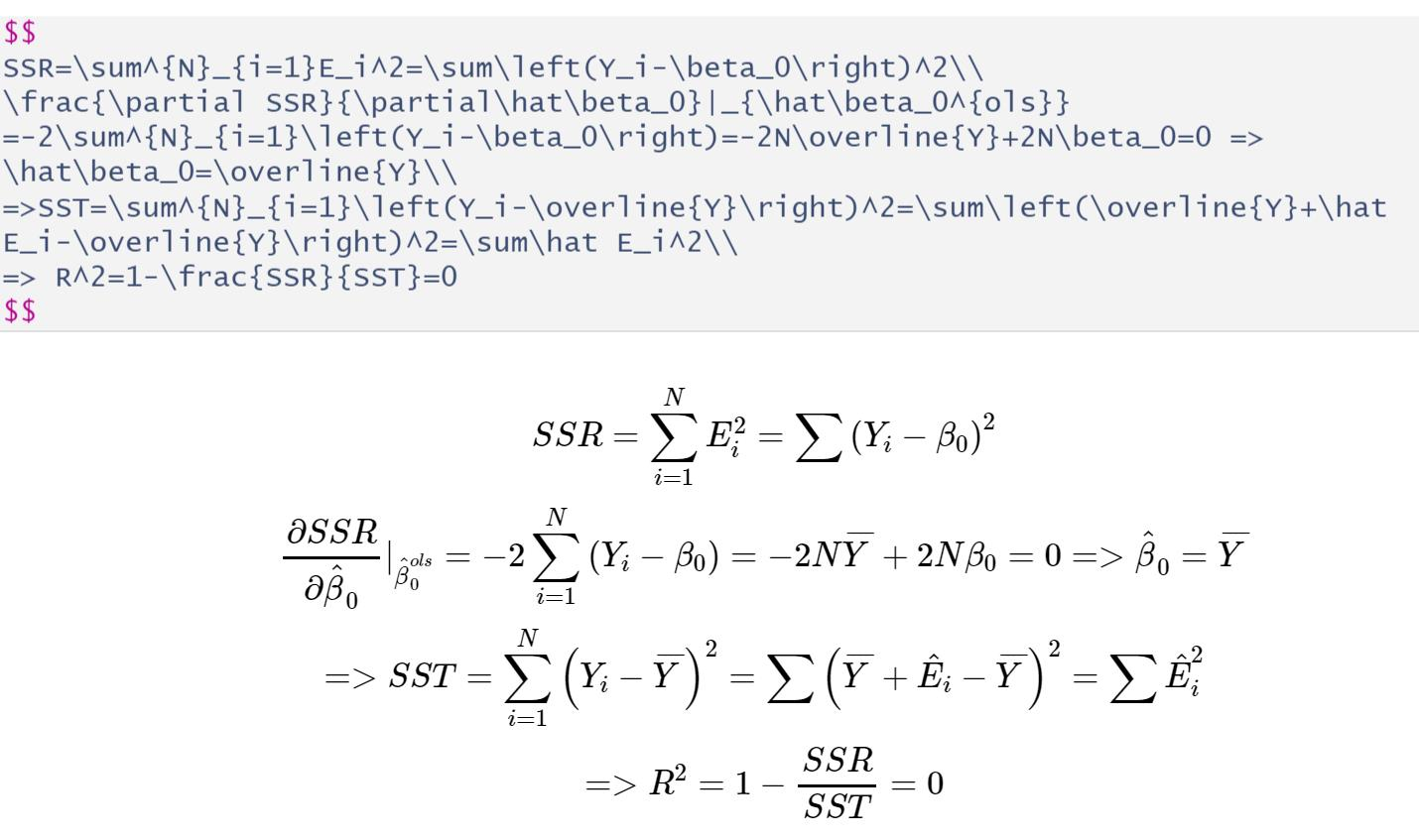SSR=\sum^{N}_{i=1}E_i^2=\sum\left(Y_i-\beta_0\right)^2\\
\frac{\partial SSR}{\partial\hat\beta_0}|_{\hat\beta_0^{ols}} =-2\sum^{N}_{i=1}\left(Y_i-\beta_0\right)=-2N\overline{Y}+2N\beta_0=0 => \hat\beta_0=\overline{Y}\\
=>SST=\sum^{N}_{i=1}\left(Y_i-\overline{Y}\right)^2=\sum\left(\overline{Y}+\hat E_i-\overline{Y}\right)^2=\sum\hat E_i^2\\
=> R^2=1-\frac{SSR}{SST}=0http://blog.fens.me/r-markdown-math-latex/This entry was posted in R语言实践

Article Rating
Subscribe
Notify ofThis site uses Akismet to reduce spam. Learn how your comment data is processed.